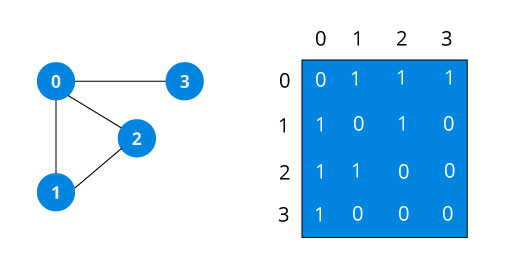An adjacency matrix is a way of representing a graph G = {V, E} as a matrix of booleans.

The size of the matrix is `VxV` where `V` is the number of vertices in the graph and the value of an entry `Aij` is either 1 or 0 depending on whether there is an edge from vertex i to vertex j.

The image below shows a graph and its equivalent adjacency matrix.In case of undirected graph, the matrix is symmetric about the diagonal because of every edge `(i,j)`, there is also an edge `(j,i)`.

The basic operations like adding an edge, removing an edge and checking whether there is an edge from vertex i to vertex j are extremely time efficient, constant time operations.

If the graph is dense and the number of edges is large, adjacency matrix should be the first choice. Even if the graph and the adjacency matrix is sparse, we can represent it using data structures for sparse matrix.

The biggest advantage however, comes from the use of matrices. The recent advances in hardware enable us to perform even expensive matrix operations on the GPU.

By performing operations on the adjacent matrix, we can get important insights into the nature of the graph and the relationship between its vertices.

The `VxV` space requirement of adjacency matrix makes it a memory hog. Graphs out in the wild usually don't have too many connections and this is the major reason why adjacency lists are the better choice for most tasks.

While basic operations are easy, operations like `inEdges` and `outEdges` are expensive when using the adjacency matrix representation.

If you know how to create two dimensional arrays, you also know how to create adjacency matrix.

``````#include <iostream>
using namespace std;

class Graph {
private:
int numVertices;
public:
Graph(int numVertices) {
this->numVertices = numVertices;
for (int i = 0; i < numVertices; i++) {
for (int j = 0; j < numVertices; j++)
}
}

void addEdge(int i, int j) {
}

void removeEdge(int i, int j) {
}

bool isEdge(int i, int j) {
}

void toString() {
for (int i = 0; i < numVertices; i++) {
cout << i << " : ";
for (int j = 0; j < numVertices; j++)
cout << adjMatrix[i][j] << " ";
cout << "\n";
}

}

~Graph() {
for (int i = 0; i < numVertices; i++)
}
};

int main(){
Graph g(4);

g.toString();
/* Outputs
0: 0 1 1 0
1: 1 0 1 0
2: 1 1 0 1
3: 0 0 1 0
*/

}``````

``````public class Graph {
private int numVertices;

public Graph(int numVertices) {
this.numVertices = numVertices;
}

public void addEdge(int i, int j) {
}

public void removeEdge(int i, int j) {
}

public boolean isEdge(int i, int j) {
}

public String toString() {
StringBuilder s = new StringBuilder();
for (int i = 0; i < numVertices; i++) {
s.append(i + ": ");
for (boolean j : adjMatrix[i]) {
s.append((j?1:0) + " ");
}
s.append("\n");
}
return s.toString();
}

public static void main(String args[])
{
Graph g = new Graph(4);

System.out.print(g.toString());
/* Outputs
0: 0 1 1 0
1: 1 0 1 0
2: 1 1 0 1
3: 0 0 1 0
*/
}

}``````

``````class Graph(object):
def __init__(self, size):
for i in range(size):
self.size = size

if v1 == v2:
print("Same vertex %d and %d" % (v1, v2))

def removeEdge(self, v1, v2):
print("No edge between %d and %d" % (v1, v2))
return

def containsEdge(self, v1, v2):
return True if self.adjMatrix[v1][v2] > 0 else False

def __len__(self):
return self.size

def toString(self):
for val in row:
print('{:4}'.format(val)),
print

def main():
g = Graph(5)